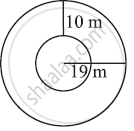Share

# Find the Circumference of the Inner and the Outer Circles, Shown in the Adjoining Figure? (Take π = 3.14) - CBSE Class 7 - Mathematics

ConceptCircle Circumference of a Circle

#### Question

Find the circumference of the inner and the outer circles, shown in the adjoining figure? (Take π = 3.14)#### Solution

Radius of outer circle = 19 m

Circumference = 2πr = 2 × 3.14 × 19 = 119.32 m

Radius of inner circle = 19 − 10 = 9 m

Circumference = 2π= 2 × 3.14 × 9 = 56.52 m

Is there an error in this question or solution?

#### APPEARS IN

NCERT Solution for Mathematics for Class 7 (2018 to Current)
Chapter 11: Perimeter and Area
Ex. 11.30 | Q: 15 | Page no. 224
Solution Find the Circumference of the Inner and the Outer Circles, Shown in the Adjoining Figure? (Take π = 3.14) Concept: Circle - Circumference of a Circle.
S